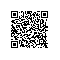# 《从缺陷中学习C/C++》——6.8　指针释放后再次使用

### 6.8　指针释放后再次使用

class　MyClass{
private:
int m_val;
public:
MyClass(int value){
m_val = value;
printf("new class A\n");
}
void getValue(){
printf("%d\n",m_val);
}
};
void function_a()
{
MyClass *A = new MyClass (1);
function_b(A);
function_c(A);
}
void function_b(MyClass *& A)
{
MyClass *B = new MyClass(2);
A->getValue();
B->getValue();
delete A;
A = NULL;
delete B;
B = NULL;}
void function_c(MyClass *&A){
A->getValue();
}
int main()
{
function_a();
return 0;
}


Bug分析

void function_a()
{
MyClass *A = new MyClass (1);
function_b(A);
function_c(A);
delete A;
A = NULL;
}
void function_b(MyClass *& A)
{
MyClass *B = new MyClass (2);
A->getValue();
B->getValue();
delete B;
B = NULL;
}
void function_c(MyClass *&A){
A->getValue();
}
int main()
{
function_a();
return 0;
}使用钉钉扫一扫加入圈子
+ 订阅